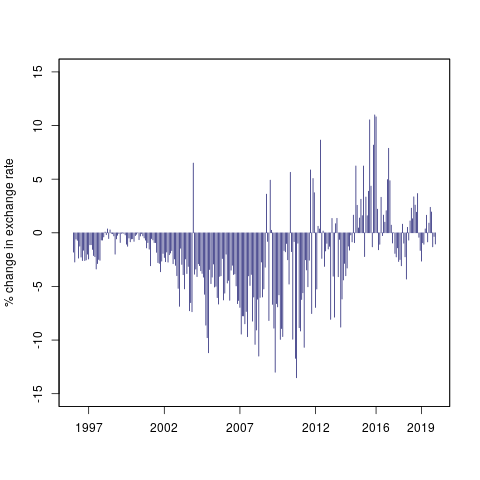# Macro/Finance Group, NIPFP

## An exchange market pressure measure for cross country analysis

Patnaik, Ila, Joshua Felman, and Ajay Shah. "An exchange market pressure measure for cross country analysis." Journal of International Money and Finance, 73 (2017): 62-77

Desai, Mohit, Ila Patnaik, Joshua Felman, and Ajay Shah. "A cross-country Exchange Market Pressure (EMP) Dataset." Data in Brief (2017)

 EMP measures in the existing literature are oriented towards applications in crisis dating and prediction. We propose a modified EMP measure where cross-country comparisons are possible. This is the sum of the observed change in the exchange rate with an estimated counterfactual of the magnitude of the change in the exchange rate associated with the observed currency intervention. We construct a multi-country dataset for EMP in each month. This opens up many new research possibilities.

• Article in Journal of International Money and Finance

• Article in Data in Brief

### Code

To get working with the dataset, here's a short demo R code for loading the dataset and plotting the EMP values for any of the 135 countries in our dataset:

```## 1) Read the EMP dataset
library(zoo)

## 2) Split the dataset by country name
emp.dat.list <- split(emp.dat,emp.dat\$country)

## 3) Country names are stored as ISO alpha 2 code of the country followed by ".curr". For example - China is stored as "cn.curr". Now we get the 2 letter country code for China
library(ISOcodes)
## Load the dataset of country code
data(ISO_3166_1)
china_cocode <- paste(tolower(ISO_3166_1[grep("^China\$",ISO_3166_1\$Name),"Alpha_2"]),".curr",sep="")

## 4) Data for EMP values of China
china.emp <- emp.dat.list[[china_cocode]]
china.emp <- zoo(china.emp[,-1],as.Date(china.emp[,1]))

## 5) Plot China EMP
plot(china.emp\$curr.emp,ylab="% change in exchange rate",xlab="",type="h",col="midnight blue",ylim=c(-15,15),xaxt = "n")
axis(1, labels = c("1997","2002","2007","2012","2018"), at = c(as.Date("1997-01-01"),as.Date("2002-01-01"),as.Date("2007-01-01"),as.Date("2012-01-01"),as.Date("2018-01-01")))

```

The above code yields the following graph for China:Figure 1: Exchange Market Pressure (EMP) values for China